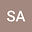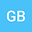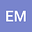On Conformable Double Sumudu Transform
•••• suliman alfaqeih,
• Gizel bakicierler,
• Emine Misirli
suliman alfaqeih
Ege Universitesi
Author ProfileGizel bakicierler
Ege University
Author ProfileEmine Misirli
Ege University
Author Profile## Abstract

In the current work, we have introduced a new deformation and generalization of the Sumudu transform called the Conformable Double Sumudu Transform (CDST), which can be applied to solve conformable fractional partial differential equations (CFPDEs). Further, we proposed some fundamental properties of the (CDST). To show the efficiency, applicability, high accuracy, and simplicity of the proposed transform, we apply this new transform to solve a wide class of linear fractional partial differential equations in the sense of Conformable fractional derivative (CFD).